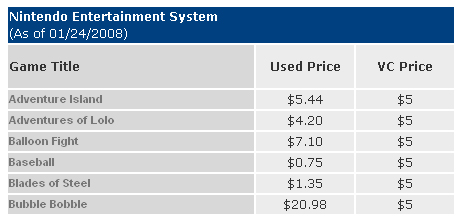NES Games
TOTAL COST – USED: \$273.53
TOTAL COST – VC: \$288.00

SNES Games
TOTAL COST – USED: \$354.38
TOTAL COST – VC: \$273.53

N64 Games
TOTAL COST – USED: \$169.42
TOTAL COST – VC: \$102.00

GENESIS Games
TOTAL COST – USED: \$406.59
TOTAL COST – VC: \$353.00

NEO GEO Games
TOTAL COST – USED: \$135.00
TOTAL COST – VC: \$72

TURBOGRAFX-16 Games
TOTAL COST – USED: \$1177.67
TOTAL COST – VC: \$294.00

TOTALS
TOTAL COST – USED: \$2516.59
TOTAL COST – VC: \$1325.00